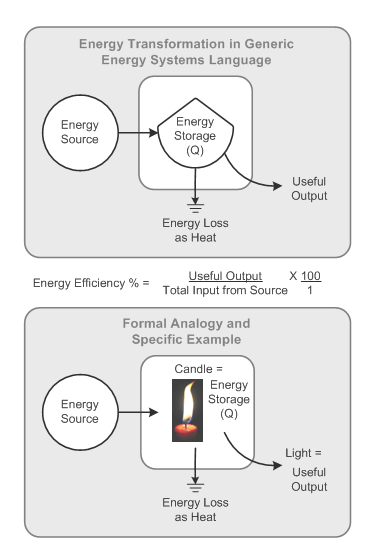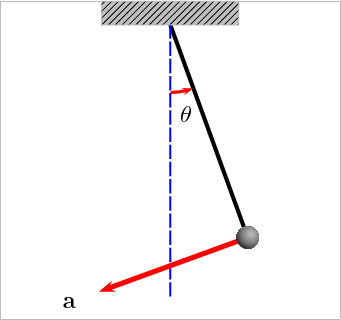# 8.24: Further Topics

$$\newcommand{\vecs}{\overset { \rightharpoonup} {\mathbf{#1}} }$$ $$\newcommand{\vecd}{\overset{-\!-\!\rightharpoonup}{\vphantom{a}\smash {#1}}}$$$$\newcommand{\id}{\mathrm{id}}$$ $$\newcommand{\Span}{\mathrm{span}}$$ $$\newcommand{\kernel}{\mathrm{null}\,}$$ $$\newcommand{\range}{\mathrm{range}\,}$$ $$\newcommand{\RealPart}{\mathrm{Re}}$$ $$\newcommand{\ImaginaryPart}{\mathrm{Im}}$$ $$\newcommand{\Argument}{\mathrm{Arg}}$$ $$\newcommand{\norm}{\| #1 \|}$$ $$\newcommand{\inner}{\langle #1, #2 \rangle}$$ $$\newcommand{\Span}{\mathrm{span}}$$ $$\newcommand{\id}{\mathrm{id}}$$ $$\newcommand{\Span}{\mathrm{span}}$$ $$\newcommand{\kernel}{\mathrm{null}\,}$$ $$\newcommand{\range}{\mathrm{range}\,}$$ $$\newcommand{\RealPart}{\mathrm{Re}}$$ $$\newcommand{\ImaginaryPart}{\mathrm{Im}}$$ $$\newcommand{\Argument}{\mathrm{Arg}}$$ $$\newcommand{\norm}{\| #1 \|}$$ $$\newcommand{\inner}{\langle #1, #2 \rangle}$$ $$\newcommand{\Span}{\mathrm{span}}$$$$\newcommand{\AA}{\unicode[.8,0]{x212B}}$$

learning objectives

• Compare the different forms of energy interrelate to one another

## Other Forms of Energy

Thermal, chemical, electric, radiant, nuclear, magnetic, elastic, sound, mechanical, luminous, and mass are forms that energy can exist in. Energy can come in a variety of forms. These forms include:

• Thermal Energy: This is energy associated with the microscopic random motion of particles in the media under consideration. An example of something that stores thermal energy is warm bath water.
• Chemical Energy: This is energy due to the way that atoms are arranged in molecules and various other collections of matter. An example of something that stores chemical energy is food. When your body digests and metabolizes food it utilizes its chemical energy.
• Electric Energy: This is energy that is from electrical potential energy, a result of Coulombic forces. Electrical potential energy is associated with the way that point charges in a system are arranged. An example of something that stores electric energy is a capacitor. A capacitor collects positive charge on one plate and negative charge on the other plate. Energy is thus stored in the resulting electrostatic field.
• Radiant Energy: This is any kind of electromagnetic radiation (see key term). An example of an electromagnetic wave is light.
• Nuclear Energy: This type of energy is liberated during the nuclear reactions of fusion and fission. Examples of things that utilize nuclear energy include nuclear power plants and nuclear weapons.
• Magnetic Energy: Technically magnetic energy is electric energy; the two are related by Maxwell’s equations. An example of something that stores magnetic energy is a superconducting magnet used in an MRI.
• Elastic Energy: This is potential mechanical energy that is stored in the configuration of a material or physical system as work is performed to distort its volume or shape. An example of something that stores elastic energy is a stretched rubber band.
• Sound Energy: This is energy that is associated with the vibration or disturbance of matter. An example of something that creates sound energy is your voice box (larynx).
• Mechanical Energy: This is energy that is associated with the motion and position of an object. It is the sum of all of the kinetic and potential energy that the object has. An example of something that utilizes mechanical energy is a pendulum.
• Luminous Energy: This is energy that can be seen because it is visible light. An example of luminous energy is light from a flashlight.
• Mass: Can be converted to energy via: $$\mathrm{E = mc^2}$$. For example, mass is converted into energy when a nuclear bomb explodes.Atomic bomb explosion: The mushroom cloud of the atomic bombing of Nagasaki, Japan

In each of the aforementioned forms, energy exists as either kinetic energy, potential energy, or a combination of both. It is important to note that the above list is not necessarily complete as we may discover new forms of energy in the future such as “dark energy. ” Also, each of the forms that energy can take on (as listed above) are not necessarily mutually exclusive. For example, luminous energy is radiant energy.

Types of Energy: A brief overview of energy, kinetic energy, gravitational potential energy, and the work-energy theorem for algebra-based physics students.

## Energy Transformations

Energy transformation occurs when energy is changed from one form to another, and is a consequence of the first law of thermodynamics.

learning objectives

• Summarize the consequence of the first law of thermodynamics on the total energy of a system

Energy transformation occurs when energy is changed from one form to another. It is a consequence of the first law of thermodynamics that the total energy of a given system can only be changed when energy is added or subtracted from the system. Often it appears that energy has been lost from a system when it simply has been transformed. For example, an internal combustion engine converts the potential chemical energy in gasoline and oxygen into heat energy. This heat energy is then converted to kinetic energy, which is then used to propel the vehicle that is utilizing the engine. The technical term for a device that converts energy from one form to another is a transducer.Energy Transformation: These figures illustrate the concepts of energy loss and useful energy output.

When analyzing energy transformations, it is important to consider the efficiency of conversion. The efficiency of conversions describes the ratio between the useful output and input of an energy conversion machine. Some energy transformations can occur with an efficiency of essentially 100%. For example, imagine a pendulum in a vacuum. As illustrated in, when the pendulum’s mass reaches its maximum height, all if its energy exists in the form of potential energy. However, when the pendulum is at its lowest point, all of its energy exists in the form of kinetic energy.Pendulum: This animation shows the velocity and acceleration vectors for a pendulum. One may note that at the maximum height of the pendulum’s mass, the velocity is zero. This corresponds to zero kinetic energy and thus all of the energy of the pendulum is in the form of potential energy. When the pendulum’s mass is at its lowest point, all of its energy is in the form of kinetic energy and we see its velocity vector has a maximum magnitude here.

Other energy transformations occur with a much lower efficiency of conversion. For example, the theoretical limit of the energy efficiency of a wind turbine (converting the kinetic energy of the wind to mechanical energy) is 59%. The process of photosynthesis is able to transform the light energy of the sun into chemical energy that can be used by a plant with an efficiency of conversion of a mere 6%.

## Potential Energy Curves and Equipotentials

A potential energy curve plots potential energy as a function of position; equipotential lines trace lines of equal potential energy.

learning objectives

• Derive the potential of a point charge

A potential energy curve plots the potential energy of an object as a function of that object’s position. For example, see. The system under consideration is a closed system, so the total energy of the system remains constant. This means that the kinetic and potential energy always have to sum to be the same value. We observe that the potential energy increases as the kinetic energy decreases and vice versa. The utility of a potential energy curve is that we can quickly determine the potential energy of the object in question at a given position.Potential Energy Curve: This figure illustrates the potential energy of a particle as a function of position. The kinetic energy is also shown and is abbreviated K.

Equipotential lines trace lines of equal potential energy. In, if you were to draw a straight horizontal line through the center, that would be an equipotential line. In and, if you travel along an equipotential line, the electric potential will be constant.Equipotential Lines for Two Equal and Opposite Point Charges: Electric field (blue) and equipotential lines (green) for two equal and opposite charges

Let us examine the physical explanation for the equipotentials lines in. The equation for the potential of a point charge is $$\mathrm{V = \frac{kQ}{r}}$$, where V is the potential, k is a constant with a value of 8.99 · 109 N m2/C2, Q is the magnitude of the point charge, and ris the distance from the charge. So, every point that is the same distance from the point charge will have the same electric potential energy. Therefore, if we draw a circle around the point charge, every point on the circle will have the same potential energy.

Work (W) is a measure of the change in potential energy $$\mathrm{(\Delta PE): W = -\DeltaPE}$$. Since the potential energy does not change along an equipotential line, you do not need to do any work to move along one. However, you do need to do work to move from one equipotential line to another. Recall that work is zero if force is perpendicular to motion; in the figures shown above, the forces resulting from the electric field are in the same direction as the electric field itself. So we note that each of the equipotential lines must be perpendicular to the electric field at every point.

## Key Points

• Thermal, chemical, electric, radiant, nuclear, magnetic, elastic, sound, mechanical, luminous, and mass are forms that energy can exist in.
• Energy exists as either kinetic energy, potential energy, or a combination of both.
• We may discover new forms of energy (like “dark energy”) in the future.
• The total energy of a given system can only be changed when energy is added or subtracted from the system.
• Often it appears that energy has been lost from a system when it simply has been transformed.
• The efficiency of conversions describes the ratio between the useful output of an energy conversion machine and the input.
• A potential energy curve plots the potential energy of an object as a function of its position.
• Equipotential lines trace lines of equal potential energy.
• You do not need to do any work to move along an equipotential line.

## Key Terms

• fusion: A nuclear reaction in which nuclei combine to form more massive nuclei with the concomitant release of energy.
• electromagnetic radiation: radiation (quantized as photons) consisting of oscillating electric and magnetic fields oriented perpendicularly to each other, moving through space
• fission: The process of splitting the nucleus of an atom into smaller particles; nuclear fission.
• pendulum: A body suspended from a fixed support so that it swings freely back and forth under the influence of gravity; it is commonly used to regulate various devices such as clocks.
• first law of thermodynamics: A version of the law of conservation of energy, specialized for thermodynamical systems. It is usually formulated by stating that the change in the internal energy of a closed system is equal to the amount of heat supplied to the system, minus the amount of work done by the system on its surroundings.
• potential energy: The energy an object has because of its position (in a gravitational or electric field) or its condition (as a stretched or compressed spring, as a chemical reactant, or by having rest mass)
• kinetic energy: The energy possessed by an object because of its motion, equal to one half the mass of the body times the square of its velocity.